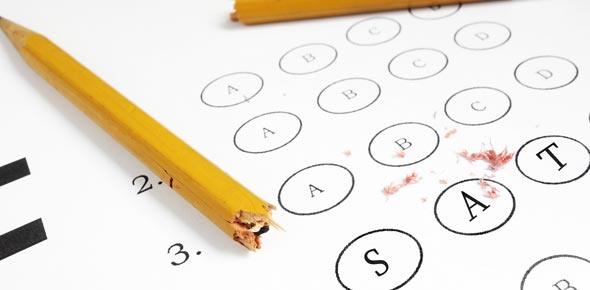# SAT 101 Part 1 Quiz For Directors

19 Questions | Total Attempts: 248SettingsRelated Topics
• 1.
• A.

Science

• B.

• C.

Math

• D.

Writing

• 2.
The SAT is used by colleges and Universities as a scholarship and financial aid tool.
• A.

True

• B.

False

• 3.
The SAT score is made up of three 800 point sections.
• A.

True

• B.

False

• 4.
What math do you not need to know for the SAT?
• A.

Calculus

• B.

Basic Arithmetic

• C.

Algebra

• D.

Geometry

• E.

All of the above

• 5.
The SAT is designed to reward Random Guessing.
• A.

True

• B.

False

• 6.
How many points are deducted for leaving a question blank on the answer sheet?
• A.

1

• B.

2

• C.

1/4

• D.

0

• 7.
How many points are deducted for choosing a wrong answer on the SAT?
• A.

1

• B.

1/4

• C.

0

• D.

1/2

• 8.
A critical reading score of 400 is considered:
• A.

Below average

• B.

Average

• C.

Above average

• D.

Top 10% of all test takers

• 9.
A score of 2050 on the SAT means that the student is in the top 10% of all test takers.
• A.

True

• B.

False

• 10.
Questions generally get easier in difficulty as you work through a section of the test.
• A.

True

• B.

False

• 11.
The obvious answer choice is always the correct choice on the SAT.
• A.

True

• B.

False

• 12.
A score of 600 on the Math section of SAT is considered:
• A.

Below average

• B.

Average

• C.

Above average

• D.

Top 10% of all test takers

• 13.
If you can eliminate at least one wrong answer choice then educated guessing can be a good strategy for the SAT.
• A.

True

• B.

False

• 14.
If a student can get 5 more questions correct on the Critical Reading Section their score generally increases:
• A.

10-20 points

• B.

40-50 points

• C.

75-100 points

• D.

150-200 points

• 15.
The key to scoring high on SAT Writing multiple choice is the ability to listen for errors.
• A.

True

• B.

False

• 16.
A longer essay usually results in a higher score on the SAT.
• A.

True

• B.

False

• 17.
Each SAT exam includes an extra 25-minute critical reading, mathematics or writing multiple-choice section that doesn’t count toward the score.
• A.

True

• B.

False

• 18.
The SAT subject tests (or SAT II tests as they are commonly referred to)  are only given on three subjects, critical reading, Math and Writing.
• A.

True

• B.

False

• 19.
All Ivy league schools require students to take and submit at least two subject tests for admission.
• A.

True

• B.

False